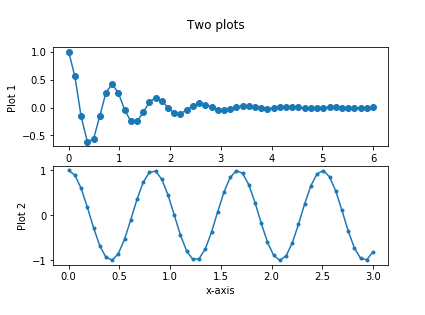# How can multiple plots be plotted in same figure using matplotlib and Python?

Matplotlib is a popular Python package that is used for data visualization.

Visualizing data is a key step since it helps understand what is going on in the data without actually looking at the numbers and performing complicated computations.

It helps in communicating the quantitative insights to the audience effectively.

Matplotlib is used to create 2 dimensional plots with the data. It comes with an object oriented API that helps in embedding the plots in Python applications. Matplotlib can be used with IPython shells, Jupyter notebook, Spyder IDE and so on.

It is written in Python. It is created using Numpy, which is the Numerical Python package in Python.

Python can be installed on Windows using the below command −

pip install matplotlib

The dependencies of Matplotlib are −

Python ( greater than or equal to version 3.4)
NumPy
Setuptools
Pyparsing
Libpng
Pytz
Free type
Six
Cycler
Dateutil

Sometimes, it may be required to understand two different data sets, one with respect to other. This is when such multiple plots can be plotted.

Let us understand how Matplotlib can be used to plot multiple plots −

## Example

import numpy as np
import matplotlib.pyplot as plt
x1_val = np.linspace(0.0, 6.0)
x2_val = np.linspace(0.0, 3.0)
y1_val = np.cos(2.3 * np.pi * x1_val) * np.exp(−x1_val)
y2_val = np.cos(2.4 * np.pi * x2_val)
fig, (ax1, ax2) = plt.subplots(2, 1)
fig.suptitle('Two plots')
ax1.plot(x1_val, y1_val ,'o−')
ax1.set_ylabel('Plot 1')
ax2.plot(x2_val, y2_val, '.−')
ax2.set_xlabel('x-axis')
ax2.set_ylabel('Plot 2')
plt.show()

## Output## Explanation

• The required packages are imported and its alias is defined for ease of use.

• The data is created using the ‘Numpy’ library for two different data sets.

• An empty figure is created using the ‘figure’ function.

• The data is plotted using the ‘plot’ function.

• The set_xlabel, set_ylabel and set_title functions are used to provide labels for ‘X’ axis, ‘Y’ axis and title.

• It is shown on the console using the ‘show’ function.

Updated on: 18-Jan-2021

183 Views